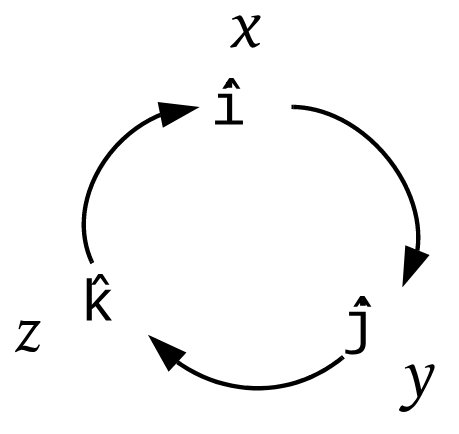# Problem: Displacement d1 is in the yz plane 63.0° from the positive direction of the y-axis, has a positive z component, and has a magnitude of 4.50 m. Displacement  d2 is in the xz plane 30.0° from the positive direction of the x-axis, has a positive z component, and has magnitude 1.40 m. What are (a)  d1 ⋅ d2(b)  d1 ⨯ d2, and (c) the angle between  d1 and d2?

🤓 Based on our data, we think this question is relevant for Professor Leuenberger's class at UCF.

###### FREE Expert Solution

This problem asks for the scalar and vector products of two vectors, as well as the angle between them.

To finda vector's components from a magnitude and angle, we use these general equations for vectors in 3D:

.

Dot Product of Two Vectors. Whenever we're asked to find the dot product of two vectors, we'll use one of these two equations:

(the definition of dot product) or

$\overline{)\stackrel{\mathbf{⇀}}{\mathbit{A}}{\mathbf{·}}\stackrel{\mathbf{⇀}}{\mathbit{B}}{\mathbf{=}}{{\mathbit{A}}}_{{\mathbit{x}}}{{\mathbit{B}}}_{{\mathbit{x}}}{\mathbf{+}}{{\mathbit{A}}}_{{\mathbit{y}}}{{\mathbit{B}}}_{{\mathbit{y}}}{\mathbf{+}}{{\mathbit{A}}}_{{\mathbit{z}}}{{\mathbit{B}}}_{{\mathbit{z}}}}$.

In most cases, using the component form (the second equation) is easiest, because it's simpler than finding θ if the vectors are 3D or given in components.

Cross Product of Two Vectors. To calculate the cross product of two vectors, it's almost always easiest to use the components. You might remember that it's a long, complicated-looking equation. To make it easier to remember, we use this circle of unit-vectors and the directions they go with:We'll show in part (b) how to use it!

Angle Between Two Vectors. When we have information about both the dot product and the cross product of two vectors, we can calculate the angle between them using the definition of dot product and the equation for the magnitude of the cross product, which is:

Dividing one equation by the other:

If we have the cross product in unit-vector form, we calculate its magnitude using the equation

$\overline{){\mathbf{|}}\stackrel{\mathbf{⇀}}{\mathbit{A}}{\mathbf{|}}{\mathbf{=}}\sqrt{{{\mathbit{A}}_{\mathbit{x}}}^{\mathbf{2}}\mathbf{+}{{\mathbit{A}}_{\mathbit{y}}}^{\mathbf{2}}\mathbf{+}{{\mathbit{A}}_{\mathbit{z}}}^{\mathbf{2}}}}$###### Problem Details

Displacement d1 is in the yz plane 63.0° from the positive direction of the y-axis, has a positive z component, and has a magnitude of 4.50 m. Displacement  d2 is in the xz plane 30.0° from the positive direction of the x-axis, has a positive z component, and has magnitude 1.40 m. What are

(a)  d1d2

(b)  d1d2, and

(c) the angle between  d1 and d2?